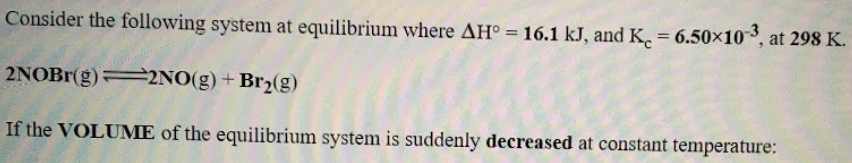# Consider the following system at equilibrium where ΔH° = 16.1 kJ, and Kc = 6.50x10^-3, at 298 K. 2NOBr(g) ⇌ 2NO(g) + Br2(g) If the VOLUME of the equilibrium system is suddenly decreased at constant temperature: The value of Qc a) is greater than Kc. b) is equal to Kc c) is less than Kc.